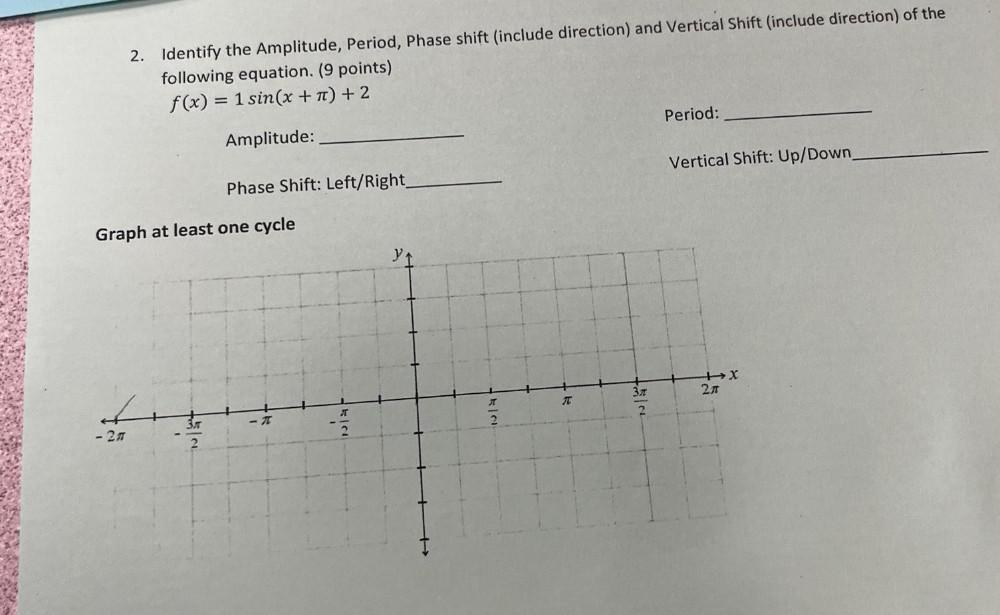Question:

# Identify the Amplitude, Period, Phase shift (include direction) and Vertical Shift (include direction) of the following equation. f(x) = 1 sin(x + π) + 2Identify the Amplitude, Period, Phase shift (include direction) and Vertical Shift (include direction) of the following equation. f(x) = 1 sin(x + π) + 2